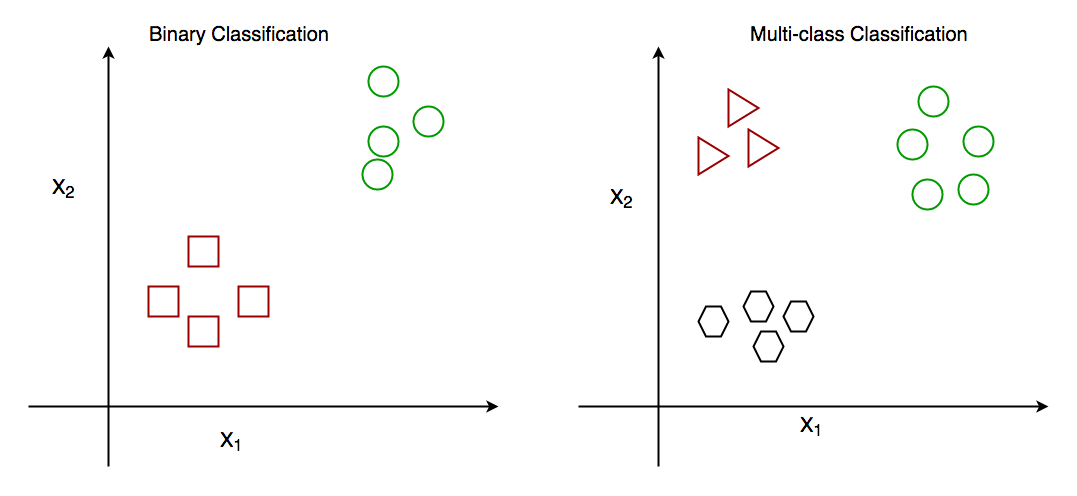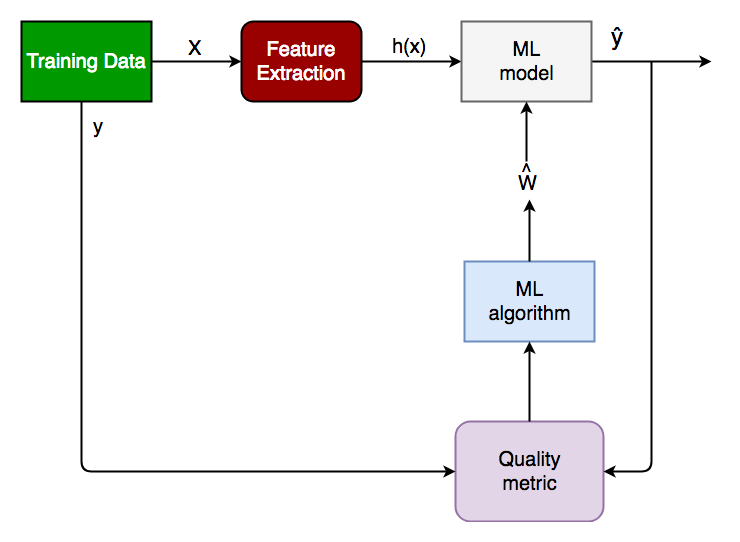# Getting started with Classification

• Difficulty Level : Easy
• Last Updated : 15 Jun, 2022

As the name suggests, Classification is the task of “classifying things” into sub-categories. But, by a machine! If that doesn’t sound like much, imagine your computer being able to differentiate between you and a stranger. Between a potato and a tomato. Between an A grade and an F. Now, it sounds interesting now. In Machine Learning and Statistics, Classification is the problem of identifying to which of a set of categories (subpopulations), a new observation belongs, on the basis of a training set of data containing observations and whose categories membership is known.

### Types of Classification

Classification is of two types:

1. Binary Classification: When we have to categorize given data into 2 distinct classes. Example – On the basis of given health conditions of a person, we have to determine whether the person has a certain disease or not.
2. Multiclass Classification: The number of classes is more than 2. For Example – On the basis of data about different species of flowers, we have to determine which specie our observation belongs.Fig: Binary and Multiclass Classification. Here x1 and x2 are the variables upon which the class is predicted.

### How does classification works?

Suppose we have to predict whether a given patient has a certain disease or not, on the basis of 3 variables, called features.

This means there are two possible outcomes:

1. The patient has the said disease. Basically, a result labeled “Yes” or “True”.
2. The patient is disease-free. A result labeled “No” or “False”.

This is a binary classification problem.
We have a set of observations called the training data set, which comprises sample data with actual classification results. We train a model, called Classifier on this data set, and use that model to predict whether a certain patient will have the disease or not.
The outcome, thus now depends upon :

1. How well these features are able to “map” to the outcome.
2. The quality of our data set. By quality, I refer to statistical and Mathematical qualities.
3. How well our Classifier generalizes this relationship between the features and the outcome.
4. The values of the x1 and x2.

Following is the generalized block diagram of the classification task.Generalized Classification Block Diagram.

1. X: pre-classified data, in the form of a N*M matrix. N is the no. of observations and M is the number of features
2. y: An N-d vector corresponding to predicted classes for each of the N observations.
3. Feature Extraction: Extracting valuable information from input X using a series of transforms.
4. ML Model: The “Classifier” we’ll train.
5. y’: Labels predicted by the Classifier.
6. Quality Metric: Metric used for measuring the performance of the model.
7. ML Algorithm: The algorithm that is used to update weights w’, which update the model and “learns” iteratively.

Types of Classifiers (algorithms)

There are various types of classifiers. Some of them are :

• Linear Classifiers: Logistic Regression
• Tree-Based Classifiers: Decision Tree Classifier
• Support Vector Machines
• Artificial Neural Networks
• Bayesian Regression
• Gaussian Naive Bayes Classifiers
• Stochastic Gradient Descent (SGD) Classifier
• Ensemble Methods: Random Forests, AdaBoost, Bagging Classifier, Voting Classifier, ExtraTrees Classifier

A detailed description of these methodologies is beyond an article!

Practical Applications of Classification

1. Google’s self-driving car uses deep learning-enabled classification techniques which enables it to detect and classify obstacles.
2. Spam E-mail filtering is one of the most widespread and well-recognized uses of Classification techniques.
3. Detecting Health Problems, Facial Recognition, Speech Recognition, Object Detection, Sentiment Analysis all use Classification at their core.

### Implementation:

Let’s get a hands-on experience with how Classification works. We are going to study various Classifiers and see a rather simple analytical comparison of their performance on a well-known, standard data set, the Iris data set.

Requirements for running the given script:

1. Python 3.8.10
2. Scipy and Numpy
3. Pandas for data i/o
4. Scikit-learn Provides all the classifiers

Example

## Python

 `# Python program to perform classification on Iris dataset` `# Run this program on your local Python interpreter``# provided you have installed the required libraries` `# Importing the required libraries``import` `numpy as np``import` `pandas as pd``from` `sklearn.model_selection ``import` `train_test_split``from` `sklearn.metrics ``import` `accuracy_score``from` `sklearn ``import` `datasets``from` `sklearn ``import` `svm``from` `sklearn.tree ``import` `DecisionTreeClassifier``from` `sklearn.naive_bayes ``import` `GaussianNB` `# import the iris dataset``iris ``=` `datasets.load_iris()``X ``=` `iris.data``y ``=` `iris.target` `# splitting X and y into training and testing sets``X_train, X_test, y_train, y_test ``=` `train_test_split(``    ``X, y, test_size``=``0.3``, random_state``=``1``)` `# GAUSSIAN NAIVE BAYES``gnb ``=` `GaussianNB()``# train the model``gnb.fit(X_train, y_train)``# make predcitions``gnb_pred ``=` `gnb.predict(X_test)``# print the accuracy``print``(``"Accuracy of Gaussian Naive Bayes: "``, accuracy_score(y_test, gnb_pred))` `# DECISION TREE CLASSIFIER``dt ``=` `DecisionTreeClassifier(random_state``=``0``)``# train the model``dt.fit(X_train, y_train)``# make predcitions``dt_pred ``=` `dt.predict(X_test)``# print the accuracy``print``(``"Accuracy of Decision Tree Classifier: "``, accuracy_score(y_test, dt_pred))` `# SUPPORT VECTOR MACHINE``svm_clf ``=` `svm.SVC(kernel``=``'linear'``)  ``# Linear Kernel``# train the model``svm_clf.fit(X_train, y_train)``# make predcitions``svm_clf_pred ``=` `svm_clf.predict(X_test)``# print the accuracy``print``(``"Accuracy of Support Vector Machine: "``,``      ``accuracy_score(y_test, svm_clf_pred))`

Conclusion: Classification is a very vast field of study. Even though it comprises a small part of Machine Learning as a whole, it is one of the most important ones.
That’s all for now. In the next article, we will see how Classification works in practice and get our hands dirty with Python Code.